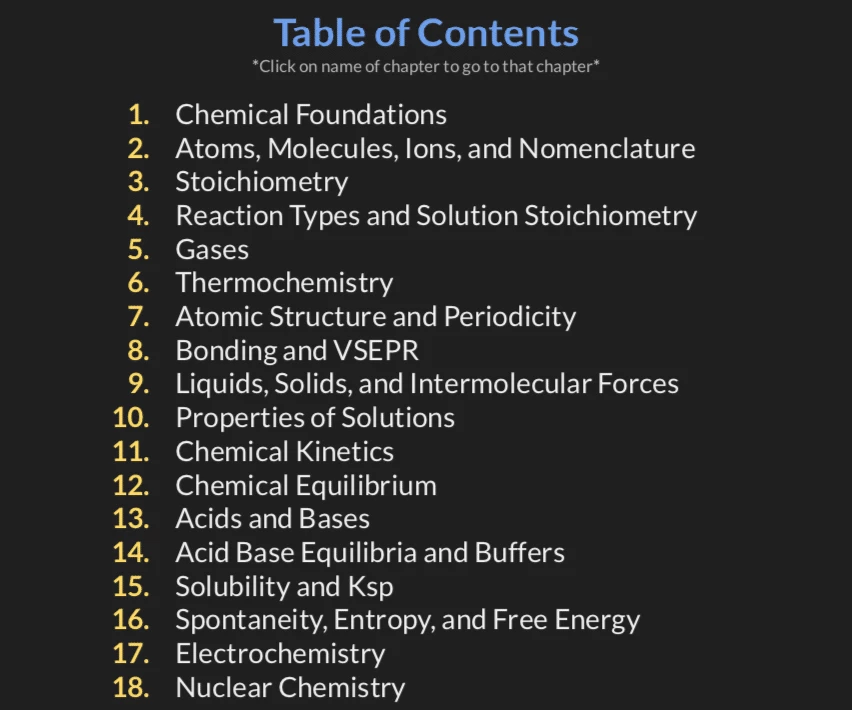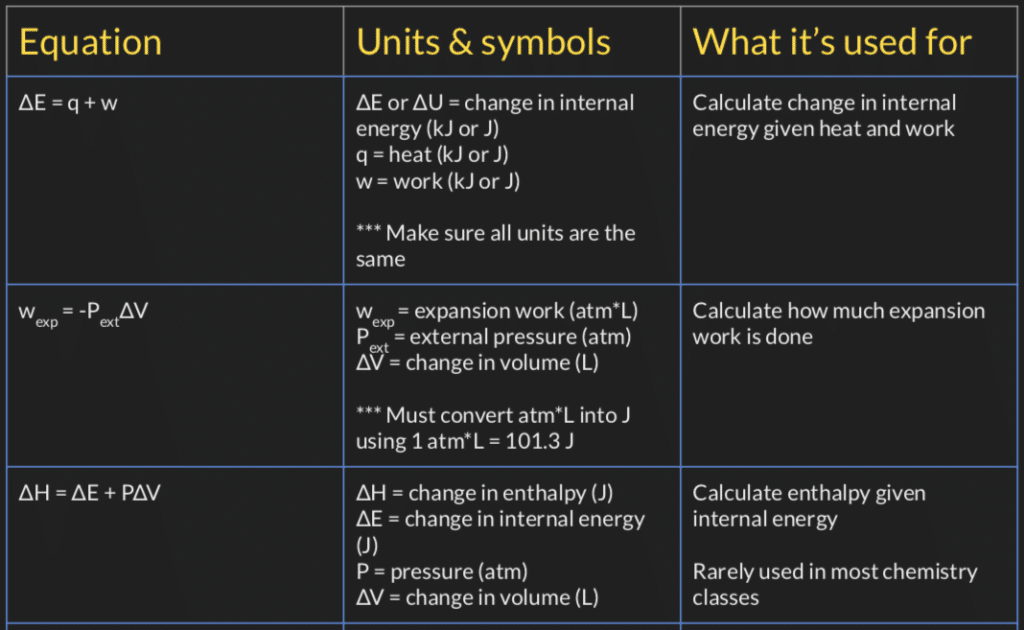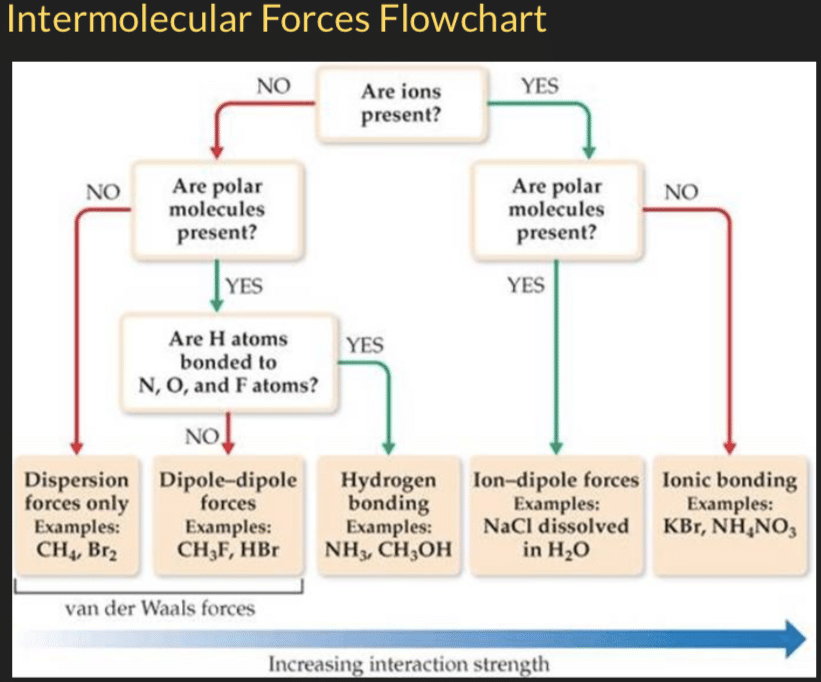Skip to content# The Ultimate Chemistry Reference Sheet and Equation Guide

\$10.00

✅ This is a DIGITAL DOWNLOAD, no physical product will be shipped.

Chemistry is filled with so many equations, constants, and rules. If you’ve ever read a question and didn’t know how to start the problem or which equation to use, then this is the perfect guide for you. If you ever wanted all the equations, constants, and rules to be in one place, then this is the guide for you.

In this 40+ page guide organized by chapters, you’ll find:

• Every single equation you’ll ever see in your chemistry class all in one place
• The meaning of the variables in each equation as well as what unit they should be in
• Explanation about the purpose of each equation as well as when to use which equation
• All the relevant conversion units
• All the constants and values you\’ll need for calculations such as Ka, Kb, Ksp, ∆H, S, ∆G, reduction potential table, etc
• Simple visuals and flowcharts for the most important skills in chemistry, such as stoichiometry, intermolecular forces, oxidation number, etc
• Shortcuts, tips, and tricks for the most commonly seen problems

With this guide, you will

• Know EXACTLY which equation to use after reading a problem
• Know what units each variable should be in
• Have a pre-made comprehensive cheat sheet & equation guide ready for all your quizzes and exams
• Learn the most important chemistry skills in a matter of seconds or minutes with straight forward easy to follow flowcharts & pictures
• Save tens to hundreds of hours by not having to look in the book or internet for equations and constant
• Be able to easily locate the information you need with the searchable function of digital documents
• Access the guide on all your electronic devices

This is the guide I wish I had when I was taking chemistry. It would have saved me so much time and made life in chemistry much easier. Get the guide today and start excelling in chemistry.